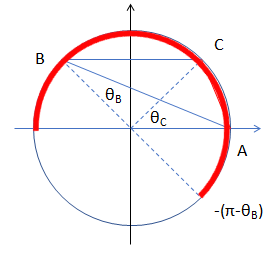#### 圆环上随机三个点组成一个锐角三角形的概率

数学 概率论 趣味数学    浏览次数：7442        分享

0Robin峰   2018-08-09 12:13

##### 4个回答
7$$\int_0^\pi {\int_{ - (\pi - {\theta _B})}^\pi {p({\theta _C})p({\theta _B})d{\theta _C}} } d{\theta _B}=\frac{1}{4{\pi^2}}\int_0^\pi({2\pi-\theta_B})d\theta_B=\frac{3}{8}$$

SofaSofa数据科学社区DS面试题库 DS面经Zealing   2018-08-10 04:14

4

SofaSofa数据科学社区DS面试题库 DS面经nobodyoo1   2018-08-09 20:45

3

$$x_1\in (0, 0.5),~~x_2 \in (0.5, 0.5+x_1)$$

$$\int_{0}^{1/2}1\int_{1/2}^{x_1+1/2}1dx_2dx_1=\int_0^{1/2}x_1dx_1=\frac{1}{4}$$

-------------------------

SofaSofa数据科学社区DS面试题库 DS面经mrhust   2018-08-27 10:43

1

SofaSofa数据科学社区DS面试题库 DS面经baolidakai   2018-08-13 18:39相关主题